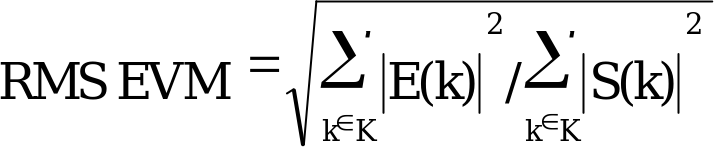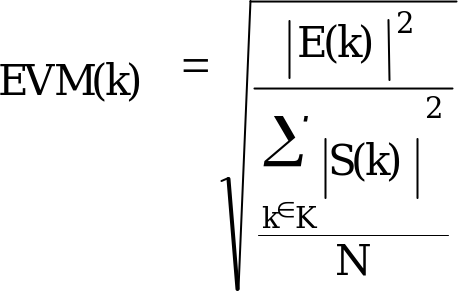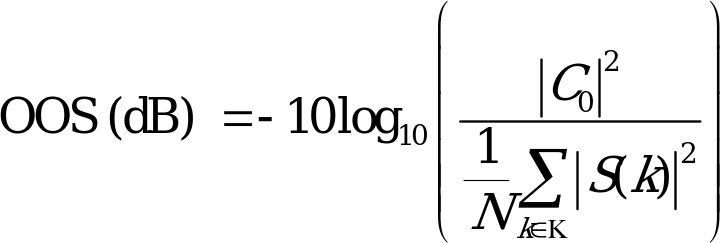## F.4 Guidelines (informative)

The specifications of the AFLC, have been developed to ensure that a generic AFLC causes minimal degradation of the parametric performance of the MS, to which it is connected.

The following should be clearly marked on the AFLC:

‑ The intended band(s) of operation.

‑ The power class of the MS, to which it designed to be connected.

When installed correctly the AFLC can provide enhancement of the MS to BTS link in vehicular installations. However, it is not guaranteed that an AFLC, which meets the requirements of this specification, will provide a performance improvement for all of the different GSM MS implementations and installations.

Some MS implementations significantly exceed the performance requirements of 3GPP TS 05.05, e.g. with respect to reference sensitivity performance. A purely passive feeder of low loss cable, may provide the best performance for some implementations. The benefits of installing an AFLC in a vehicular application, can only be assessed on a case by case basis.

When used, the AFLC should only be installed in the type approved configuration, with the minimum amount of additional cabling.

When designing an AFLC to be used with a GSM MS, the best downlink performance will be obtained if the low noise amplifier is situated as closely as possible to the output of the antenna.

Annex G (normative):
Calculation of Error Vector Magnitude

The Error Vector Magnitude (EVM) is computed at the symbol times in the useful part of the burst.
Let Y(t) be the complex signal produced by observing the real transmitter at instant t. R(t) is defined to be an ideal transmitted signal. The symbol timing phase of Y(t) is aligned with the ideal signal. The transmitter is modelled as:

Y(t) = C1{R(t) + D(t) + C0}W

where

W = e  + j accounts for both a frequency offset of “” radians per second phase rotation and an amplitude change of “” nepers per second,

C0 is a constant origin offset representing carrier feedthrough,

C1 is a complex constant representing the arbitrary phase and output power of the transmitter and

D(t) is the residual complex error on signal R(t).

Y(t) is compensated in amplitude, frequency and phase by multiplying by the complex factor

W-t/C1

After compensation, Y(t) is passed through the specified measurement filter to produce the signal

Z(k) = S(k) + E(k) + C0

where

S(k) is the ideal transmitter signal observed through the measurement filter.

k = floor(t/Ts), where Ts =1/270.833kHz corresponding to the symbol times.

The error vector E(k)

E(k) = Z(k) – C0 –S(k)

is measured and calculated for each instant k.

The sum square vector error for each component is calculated over one burst. The relative RMS vector error is defined as:and shall not exceed the specified value.

The symbol vector error magnitude (EVM) at symbol k is defined as,

where N is the number of elements in the set . EVM(k) is the vector error length relative the root average energy of the useful part of the burst.

C0, C1 and W shall be chosen to minimise RMS EVM per burst and are then used to compute the individual vector errors E(k) on each symbol. The symbol timing phase of the samples used to compute the vector error should also be chosen to give the lowest value for the RMS EVM.

Origin offset suppression (in dB) is defined asThe minimum value of origin offset suppression is specified separately.

In the above equation, the errors shall be measured after a measurement receive filter at baseband. The specification is based on using the specified, windowed, raised-cosine, filter with roll-off 0.25 and single side-band bandwidth of 90 kHz as the measurement receive filter (see 4.6.2). Sufficient over-sampling is assumed (at least 4 times).

Annex H (normative):
Requirements on Location Measurement Unit

Location Services utilizes Location Measurement Units (LMU) to support its positioning mechanisms. An LMU is additional measurement hardware in the GSM network. Time Of Arrival (TOA) positioning mechanism requires LMUs to make accurate measurement of the TOA of the access bursts emitted by the MS. Enhanced Observed Time Difference positioning mechanism requires LMUs in unsynchronized networks to measure the time difference of BTS signals received.

Section H.1 and its subsections specify LMU requirements to support the Time Of Arrival positioning mechanism.

Section H.2 and its subsections specifiy LMU requirements to support the Enhanced Observed Time Difference positioning mechanism.

An LMU may contain a control mobile station to communicate with the network. In that case, the requirements for a normal mobile station shall apply to this control mobile station.